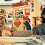## Monday, June 16, 2014

### Computing the average trait value for the set of taxa descended from each node

A recent comment asked if there was an easy way to substitute the raw average value of the tips descended from a node for ancestral states obtained via ancestral state reconstruction when using the phytools function plotBranchbyTrait. This is pretty easy. Here's a demo (although it could be done a dozen different ways, including using a simple for loop).

(This assumes only that our tree is an object of class "phylo" called tree; and, importantly, that our trait data is a named vector x, in which the names correspond to the tip labels of tree.)

getDescendants<-phytools:::getDescendants
nn<-1:tree\$Nnode+Ntip(tree)
a<-setNames(sapply(nn,function(n,x,t){
d<-getDescendants(t,n)
mean(x[t\$tip.label[d[d<=Ntip(t)]]])
},x=x,t=tree),nn)

Now we can use it with plotBranchbyTrait as follows. First, if we want each edge to be colored as the average of the parent & daughter nodes (or tip):

plotBranchbyTrait(tree,c(x,a),mode="nodes")

Alternatively, we can have the branch color determined only from the daughter nodes or tips (in which case we throw out the root value):

y<-c(setNames(x[tree\$tip.label],1:Ntip(tree)),a)
plotBranchbyTrait(tree,y[tree\$edge[,2]],mode="edges")

That's pretty much it.

#### 1 comment:

1.Thanks a lot, Liam, just what I was looking for!
Cheers,
Carsten

Note: due to the very large amount of spam, all comments are now automatically submitted for moderation.